CATEGORIES:

# Matrices and Operations on Them

Definition. A matrix of sizeis a table written in parentheses or between two pairs of parallel lines:,

m is the number of rows, n is the number of columns, and аij is an element of the matrix.

If m=n, then the matrix is square.

A null-matrix is a matrix А, in which all elements are zero: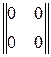;.

Definition. A square matrix А is said to be diagonal if its main diagonal may contain nonzero elements, but all nondiagonal elements are zero:.

Definition. The identity matrix is the diagonal matrix with ones on the diagonal:.

Definition. Matrices of the same size are equal if their respective elements are equal.

Two matrices of the same size can be added (elementwise):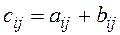.

Any matrix can be multiplied by any number all elements of the matrix should be multiplied by this number):.

Subtraction is defined as

А–В=А+(1)В.

Two matrices can be multiplied only if the number of columns in the first matrix equals the number of rows in the second matrix. An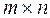matrix is multiplied by anmatrix as follows:We obtain a matrix of size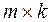.

Multiplication of matrices is not commutative, and sometimes even impossible:

АВ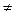ВА.

Date: 2015-01-02; view: 1091

 <== previous page | next page ==> Cramer’s Rule | The Gauss-Jordan Method
doclecture.net - lectures - 2014-2022 year. Copyright infringement or personal data (0.013 sec.)